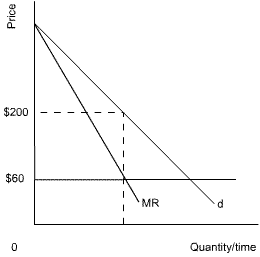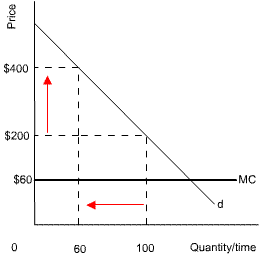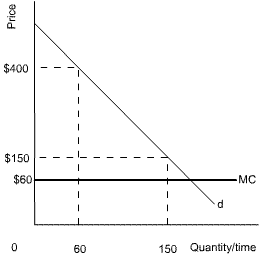### Why should I choose AnalystNotes?

AnalystNotes specializes in helping candidates pass. Period.

##### Subject 6. Price Discrimination
Price discrimination is a practice whereby a seller charges different consumers different prices for the same product or service. It converts consumer surplus into economic profit.

• In first-degree price discrimination each consumer is charged the maximum he is willing to pay. Consumer surplus is nil, while producer surplus is maximized. The output is the same as in a competitive market.
• In second-degree price discrimination, prices vary across units but not people. Consumers self-select into consumption groups and seek the largest surplus.
• In third-degree price discrimination, consumers are segregated by demographic or other traits. Prices are determined by the demands of each group.

When sellers can segment their market (at a low cost) into groups with differing price elasticities of demand, price discrimination can increase profits. For each group, the seller will maximize profit by equating marginal cost and marginal revenue. The number of units sold also increases because the discounts provided to price-sensitive groups increase the quantity sold more than the higher prices charged the less price-sensitive groups reduce sales.

Imagine that the MC per unit for a monopoly is constant at \$60, producing a horizontal MC curve, as shown below.The firm produces where MC = MR. It thus produces 100 units and charges \$200 per unit. Total revenue (price x quantity) for the firm is thus: \$200 x 100 = \$20,000. Total costs (cost per unit x quantity) are: \$60 x 100 = \$6,000. Total profit is thus: \$20,000 - \$6,000 = \$14,000.

Imagine that this firm is an airline and that it now decides to increase its profits using price discrimination. It identifies two groups of people: businessmen, who are fairly price-inelastic, and students, who are fairly price-elastic (responsive to changes in price). By increasing the price of businessmen's tickets and decreasing the price of student's tickets, it can increase its total revenue and thus increase its profit.

The airline starts by doubling the price of businessmen's tickets to \$400. By equating the businessmen's MR curve to the MC curve (for simplicity, the MR curve is not shown), the airline finds that the quantity demanded decreases, but by relatively little, given the large increase in price, to 60 tickets.Next, it equates MC to the students' MR curve and finds that it can decrease the price of students' tickets from \$200 to \$150, whilst the quantity demanded increases to 150 tickets. (Note: for simplicity, the students' MR curve is not shown).Therefore, the total revenue is as follows: \$400 x 60 + \$150 x 150 = \$46,500.
Total costs are: \$60 x 60 + \$60 x 150 = \$12,600.
Total profits are thus: \$46,500 - \$12,600 = \$33,900.

This is more than the \$14,000 profit the firm made in the absence of price discrimination.Learning Outcome Statements

f. describe pricing strategy under each market structure;

CFA® 2021 Level I Curriculum, , Volume 2, Reading 13

User Comment
bahodir Why do you think airline companies are monopolies? Aren't they, in most cases, oligopolies?
mchu Price discrimination is the practice of selling different units of a good or service for different prices. Many firms price discriminate, but not all of them are monopoly firms.
MMattioli Therefore an airline is not a monopoly, but rather an oligopoly that price discriminates
Lamkerst Price discrimination is possible in all the markets except for a price-taking one. Monopolistic competitors, oligopolies, monopolies can price-discriminate.
tomalot First example has 100 units/ customers.
Second example has 200 customers: 100 businessmen (of whom 40 walk away when price rises) and 100 students (plus 50 from the price drop)
Am I right?
blablabob Yes you are